Is pattern recognition about statistics? Well, it depends. If you see as its target to understand how new knowledge can be gained by learning from examples the role of statistics may be disputable. Knowledge lives in the human mind. It is born in the marriage between observations and reasoning. If we follow this process consciously by introspection, then statistics is not there. Human knowledge has been gained over thousands of years before we had encountered the concept of probability. So, if we would have used probabilities in gaining knowledge, we did this, at least originally,  unconsciously.

#### The entrance of statistics in PR

However, at the moment we start to analyze the process of gaining knowledge itself, and try to build a model of it, probabilities enter the scene. A good pattern recognition system, one that has gained some knowledge, is able to classify new objects accurately. Almost always this is defined by the probability of error, estimated by the fraction of wrong classifications in a test set of examples with known pattern classes.

A strong characteristic of the field of pattern recognition is that is has this very clear performance measure. It can be used to judge students in the field, to compare procedures and to organize competitions. Just using a single number that can be measured by anybody makes life easy and preferences indisputable. There are almost no studies of people who claim that their classifier might be worse in terms of numbers of mistakes, but that at the end it is better as its mistakes are less stupid. It is all about quantities. Quality plays hardly a role.

#### Probability densities and the Bayes classifier

How do we find the best classifier? It is the classifier that assigns the most probable class to new objects, given the training set of examples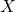. In mathematical terms, phrased by the posterior probabilities:

if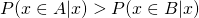then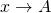elseUsing Bayes rule, the corresponding classifier can be written as: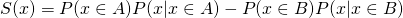, if S(x) > 0 thenelseHereand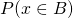are the class prior probabilities. For continuous vector representations the two other terms can be written as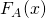and, the probability density functions of the two classes. If they reflect the class density functions in the application, and accordingly in the test set that measures the classifier performance, then this classifier is the best one possible. Because of the use of the Bayes rule in its derivation it is called the Bayes classifier and the corresponding minimum error the Bayes error.

#### Non density based classifiers

Formally the pattern recognition problem has been solved now. So why do we find many other classifiers in the literature, like the support vector machine, adaboost, random forest, decision trees and neural networks? They should be worse, or, at most, if trained very carefully, they may asymptotically approximate the Bayes classifier, under specific conditions. They are not directly based on densities, but apply them at most in a very indirect way. So why are they so popular?

The answer is straightforward, but not discussed often explicitly, so it might be good to phrase it here. Proper density estimation in high-dimensional spaces is very difficult. The dimensions of feature spaces may range from a few to many thousands. As long as the size of the training set per class is not a multiple of the dimension, the feature space is almost empty. The ‘density’ of training points in the neighborhood of a test point cannot reliably be estimated. So non-density based classifiers are of interest here.

#### Conclusion

In conclusion, classifier design is important if we just have a small training set (or want to use one for computational reasons). For larger and larger training sets their performances are expected to become at most as good as classification based on density estimation. For this reason training set sizes should be taken into account in the the study of classifier performances. In many comparative studies on classifier performances this is, unfortunately, neglected.

Filed under: ClassificationEvaluation